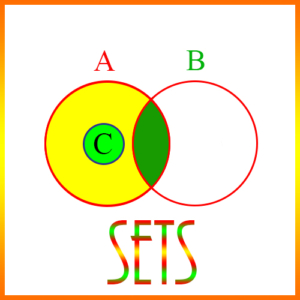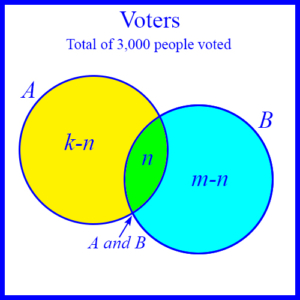# Set Theory ProblemsSet theory problems are those which use basic operations on sets. These are

• Difference of Sets
• Intersection of Sets
• Union of Sets

Associated with these operations are rules for determining the number of elements in or the cardinality of each of the resulting sets. These rules are

1. |A – B| = |A| – |A ∩ B| For the cardinality of the difference of two sets
2. |A ∪ B| = |A| + |B| – |A ∩ B| For the cardinality of the union of two sets

where A and B are sets, and |X| is the cardinality of a set X.

The following 4 versions of a single problem illustrate these operations and the calculation of cardinalities.

Version 1:

3000 people voted in a small, local election for city council. Each person could vote for 2 candidates. Candidate A got k votes, and B got m votes. Of the people who voted for either A or B, n voted for both A and B. How many people voted for either A or B?

Answer: k + m – n

Explanation: Sometimes problems like this can be confusing because of the language. When the subject is an election, and it’s said “Candidate A got k votes, B got m votes,” we are inclined to assume that votes means voters, so there is no overlap of voters for the two candidates. And we conclude that the total number of people who voted for A or B is

k + m

However, notice also that the question subtly distinguishes between votes and voters. That is, it asks how many people voted for either A or B. Since in this case, voters can vote for two candidates, it’s possible the same person could vote for both A and B, as the problem does in fact indicate – n people voted for both A and B. These ideas are illustrated in this diagram:The diagram shows how the people were divided up in the election based on the candidates they voted or did not vote for. The box is the set of 3000 voters. The two circles marked A and B represent the sets of people who voted for candidates A and B respectively. Voters who voted for both A and B are in the area where the two circles overlap. Voters outside both circles voted for neither A nor B.

Notice that the areas in the diagram are disjoint. So people in circle A but not in the overlapping area are those who voted for A but not for B. There are n people in the overlapping area, so the number of those in A but not in B is k – n, and the number who voted for B but not A is m – n.

We can get the total number of people or voters who voted for either A or B, by looking at these numbers in two different ways: (1) We can take the total number of votes the two received

k + m

and subtract out the number of people, n, who voted for both A and B, because otherwise these n people would be counted twice. Thus, the total number of people who voted for either A or B is

k + m – n

Or, (2) looking at the diagram we see that the total number is

(k – n) + n + (m – n) = (k – n + n) + m – n = k + m – n

So both methods give us the same answer, k + m – n.

The solution to this problem illustrates the use of the set union operation and calculating the cardinality of the union of two sets from the cardinalities of each of the sets individually and from that of their intersection.

Version 2:

3000 people voted in a small, local election for city council. Each person could vote for 2 candidates. Candidate A got k votes, and B got m votes. Of the people who voted for either A or B, n voted for both A and B. How many people voted for neither A nor B?

Answer: 3000 – k – m + n

Explanation: In this case we want the opposite of what we calculated in the previous problem, and the answer here uses the answer of the previous problem. Since there were 3000 voters, and since k + m – n of them voted for either A or B, then the number who voted for neither A nor B is

3000 – (k + m – n) = 3000 – k – m + n

The solution to this problem illustrates the operation of set difference, where we are taking the difference between the set of all voters and the set of voters who voted for either A or B.

Version 3:

3000 people voted in a small, local election for city council. Each person could vote for 2 candidates. Candidate A got k votes, and B got m votes. Of the people who voted for either A or B, n voted for both A and B. How many people voted for A but not for B?

Explanation: This is shown in the diagram, which for convenience is repeated here.Version 4:

3000 people voted in a small, local election for city council. Each person could vote for 2 candidates. Candidate A got k votes, and B got m votes. Of the people who voted for either A or B, n voted for both A and B. How many people voted for A or B but not for both?

Answer: k + m – 2n

Explanation: The diagram shows clearly that the number of people who voted exclusively for A or exclusively for B are k – n and m – n, respectively. Therefore, the number who voted either for A or for B, but not for both is

k – n + m – n = k + m – 2n